Learn all Concepts of Chapter 3 Class 10 (with VIDEOS). Check - Linear Equations in 2 Variables - Class 10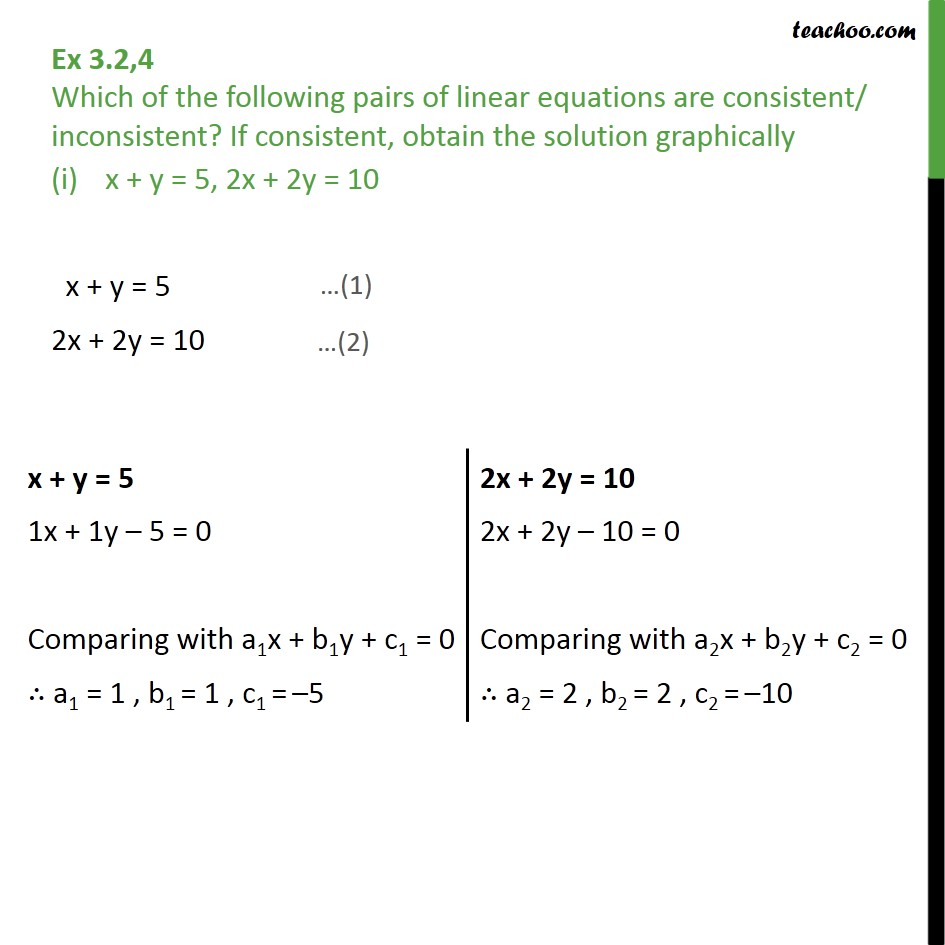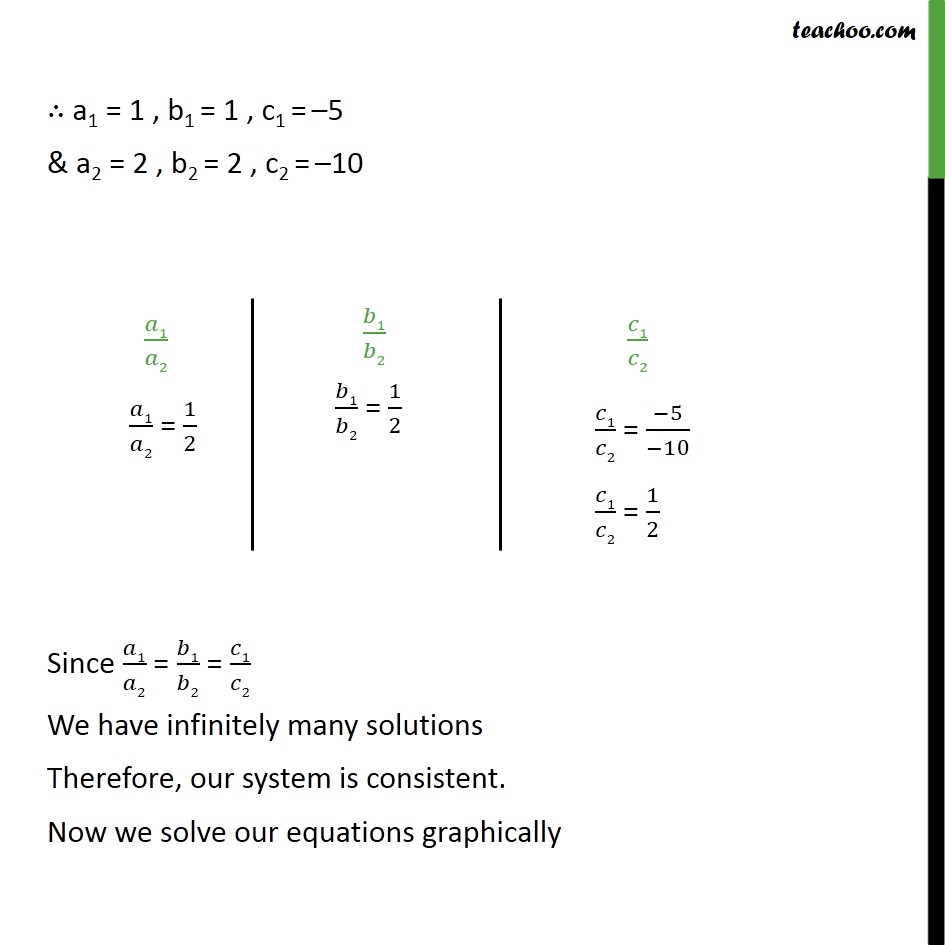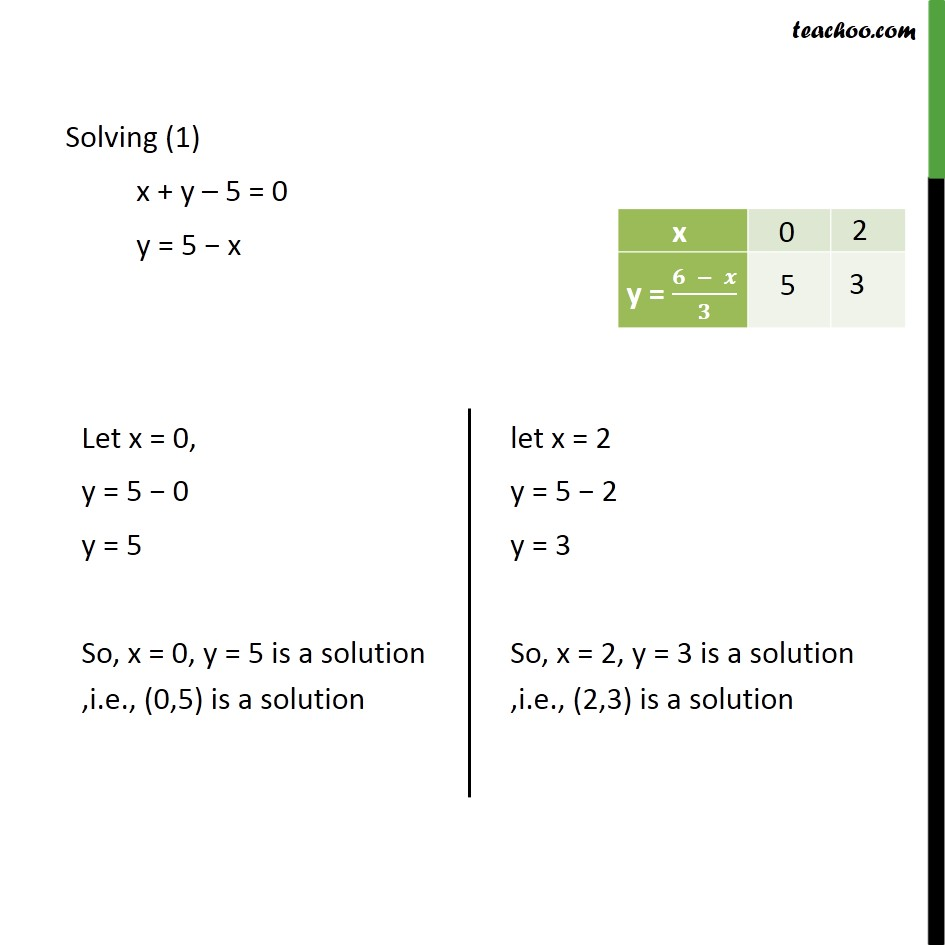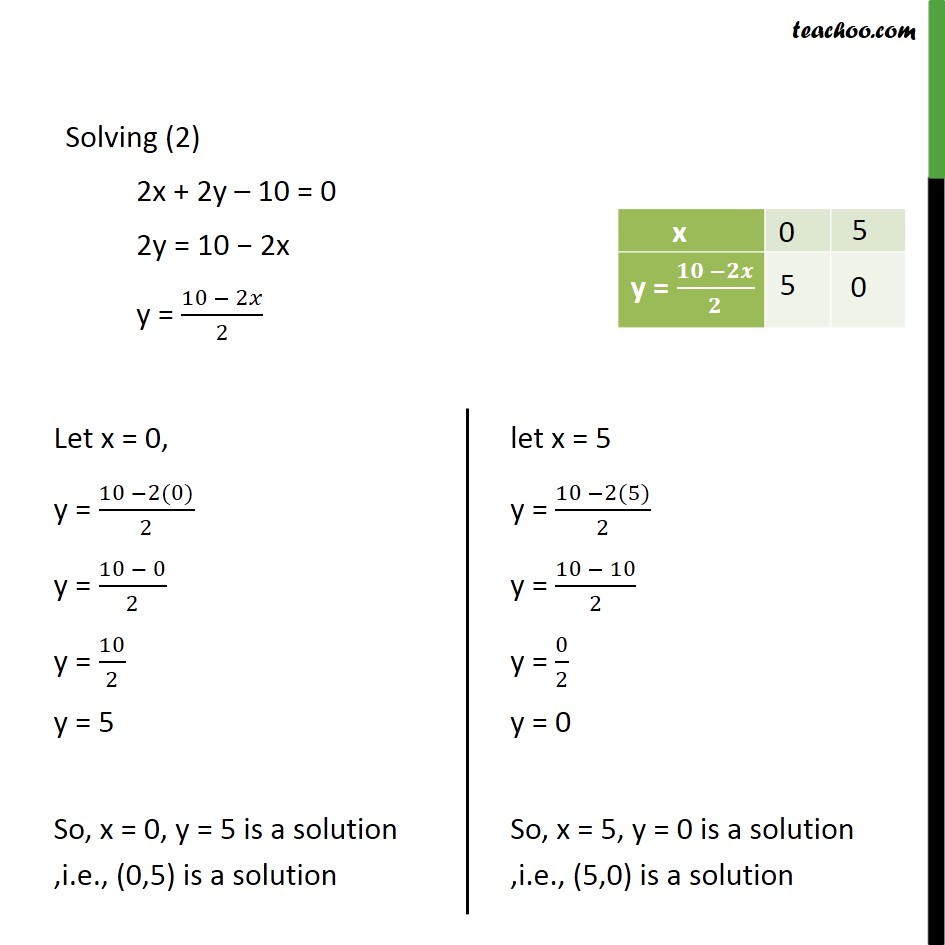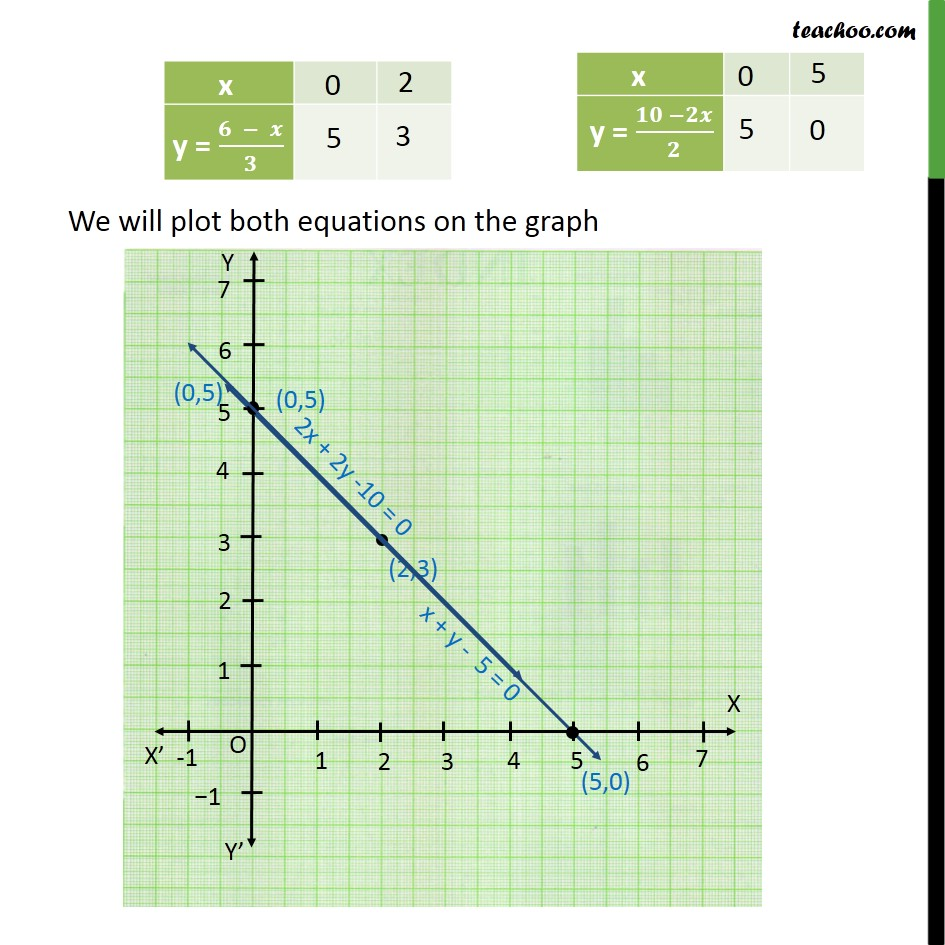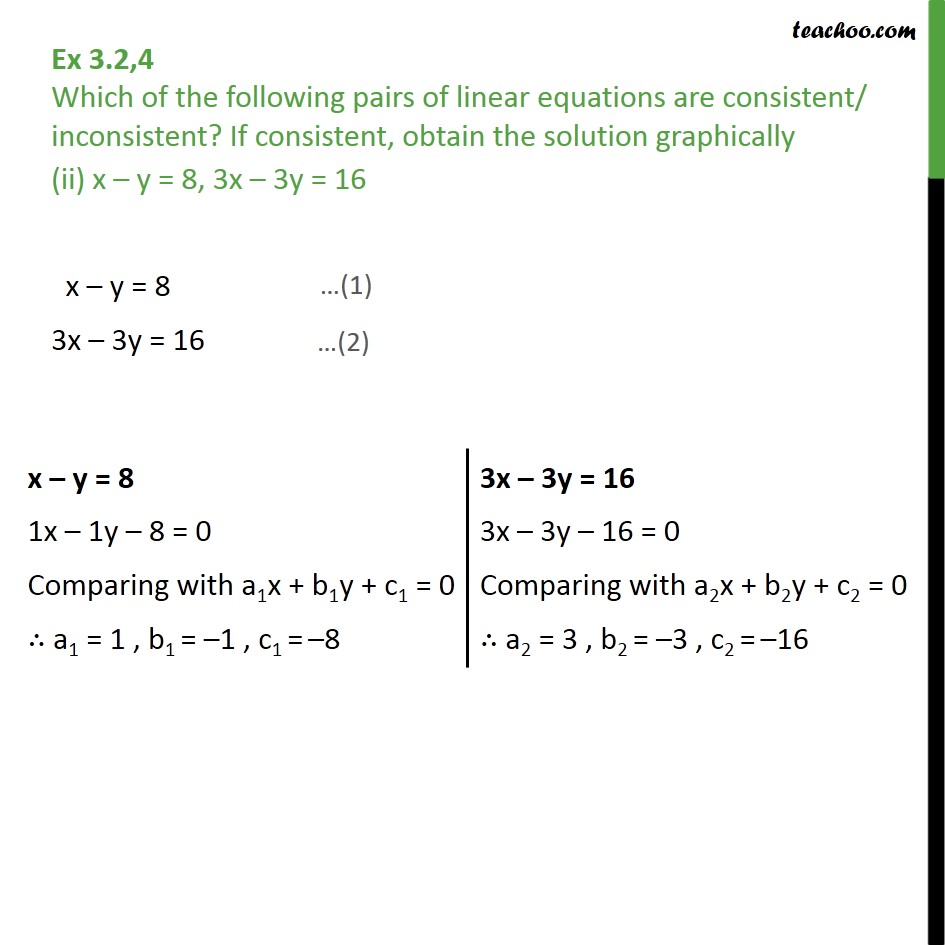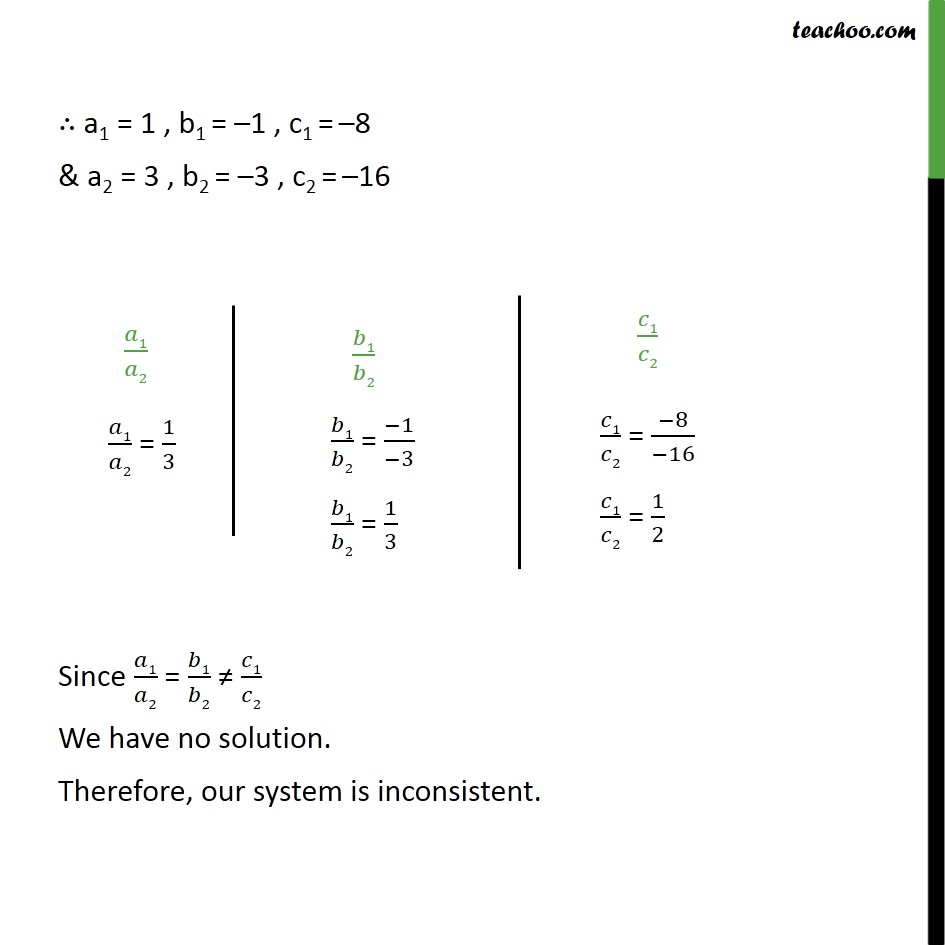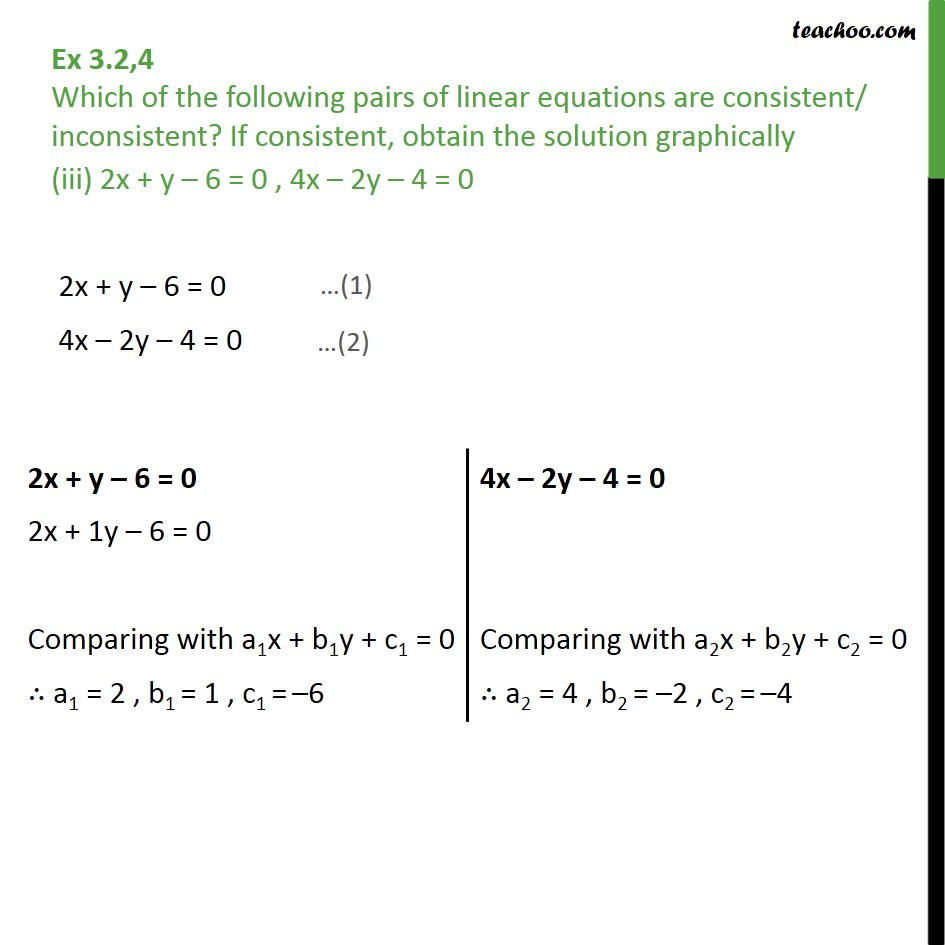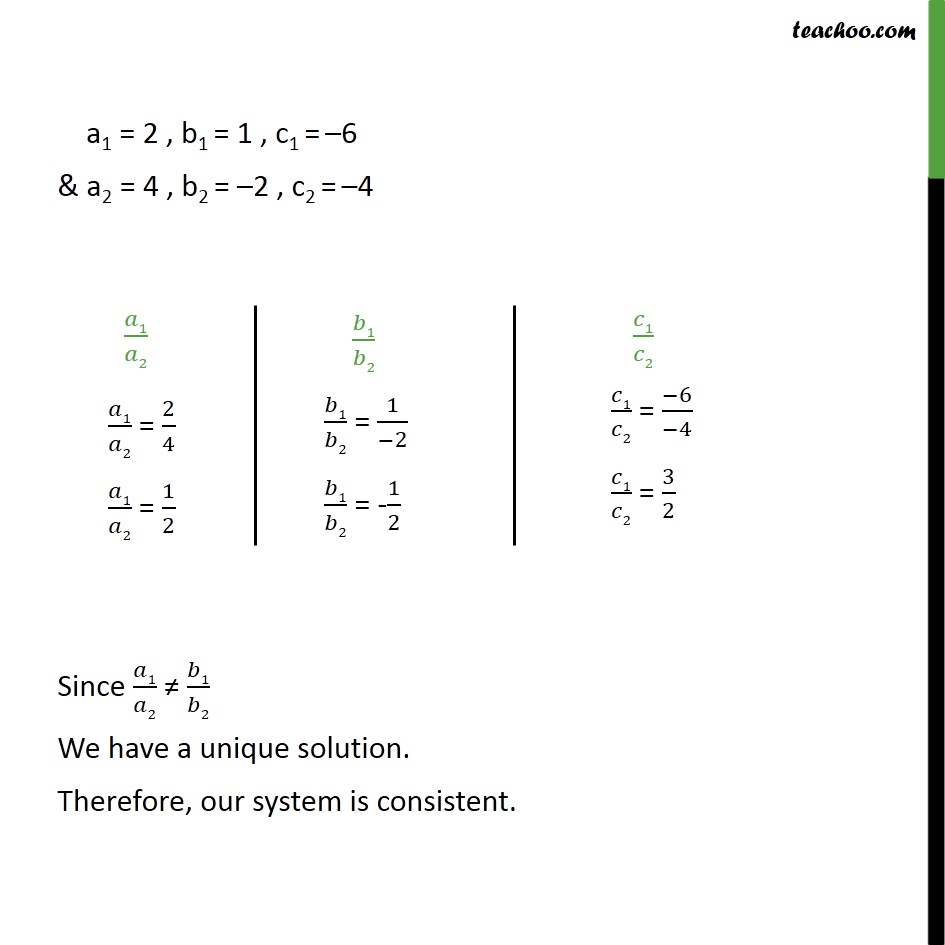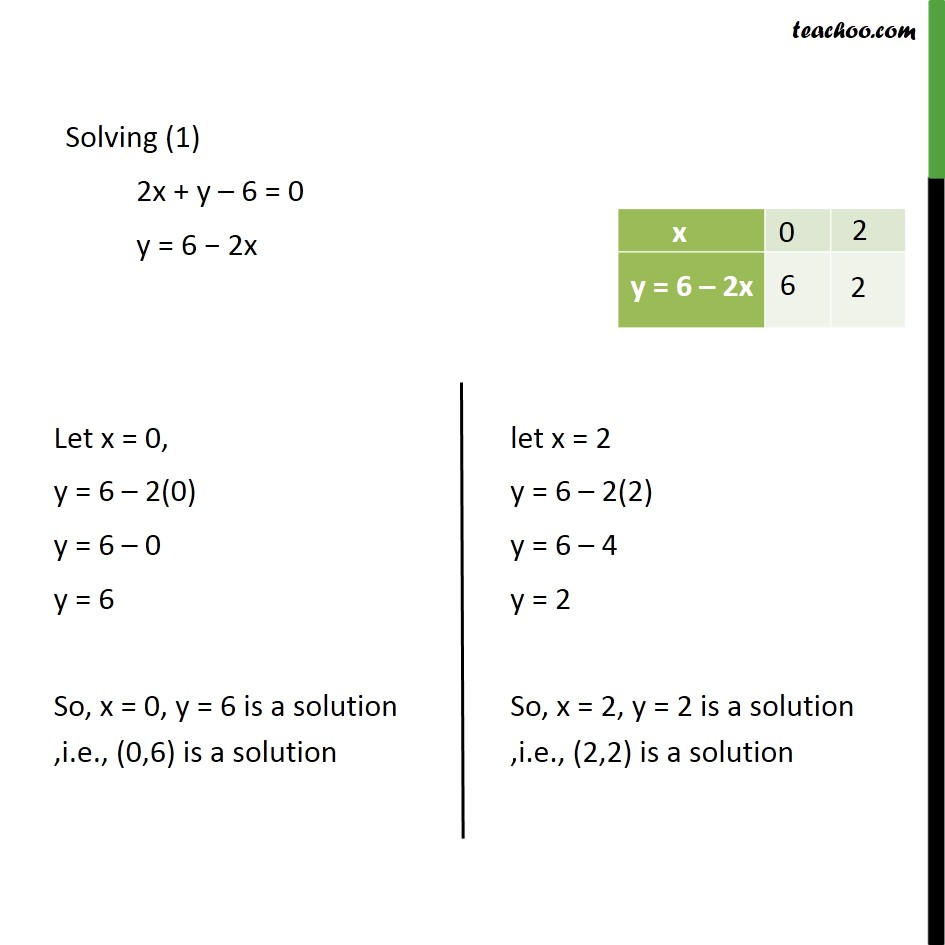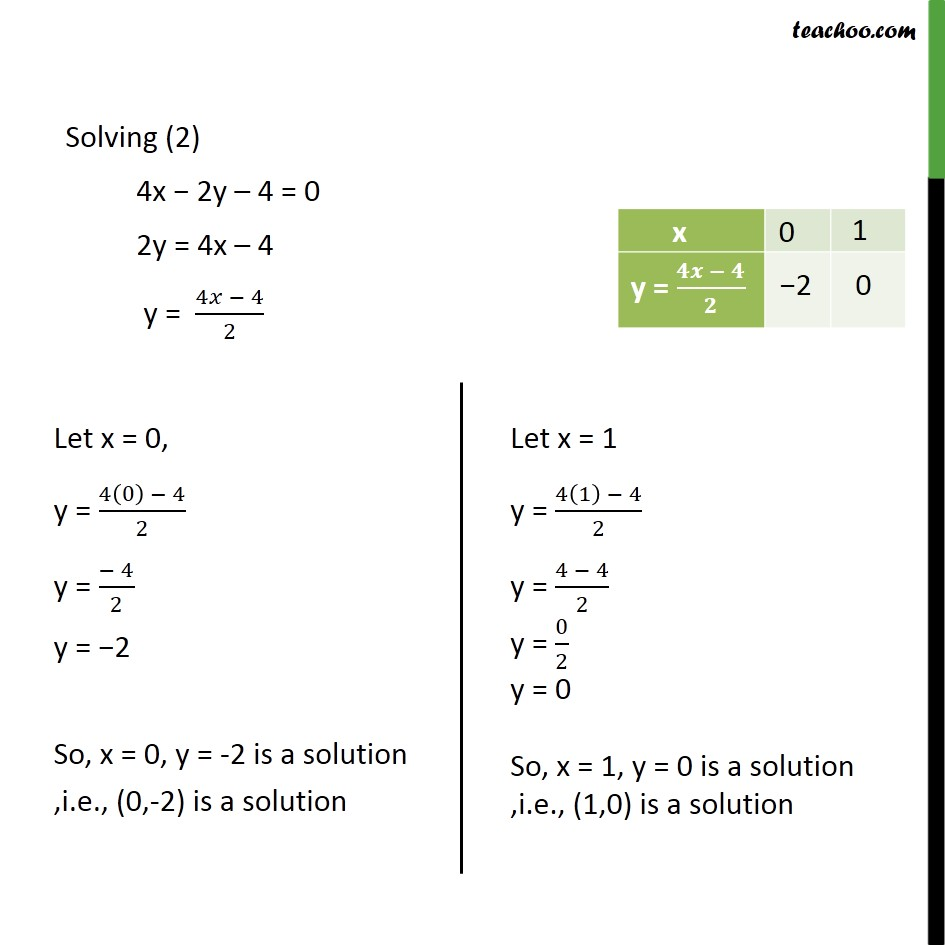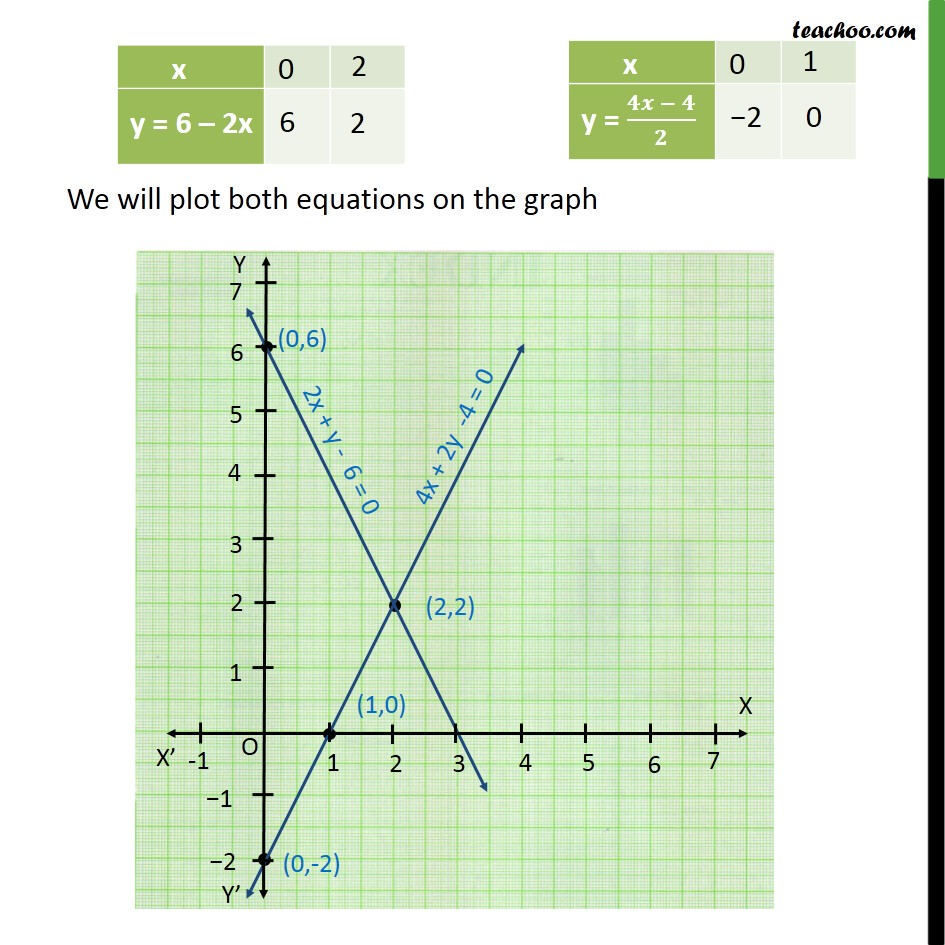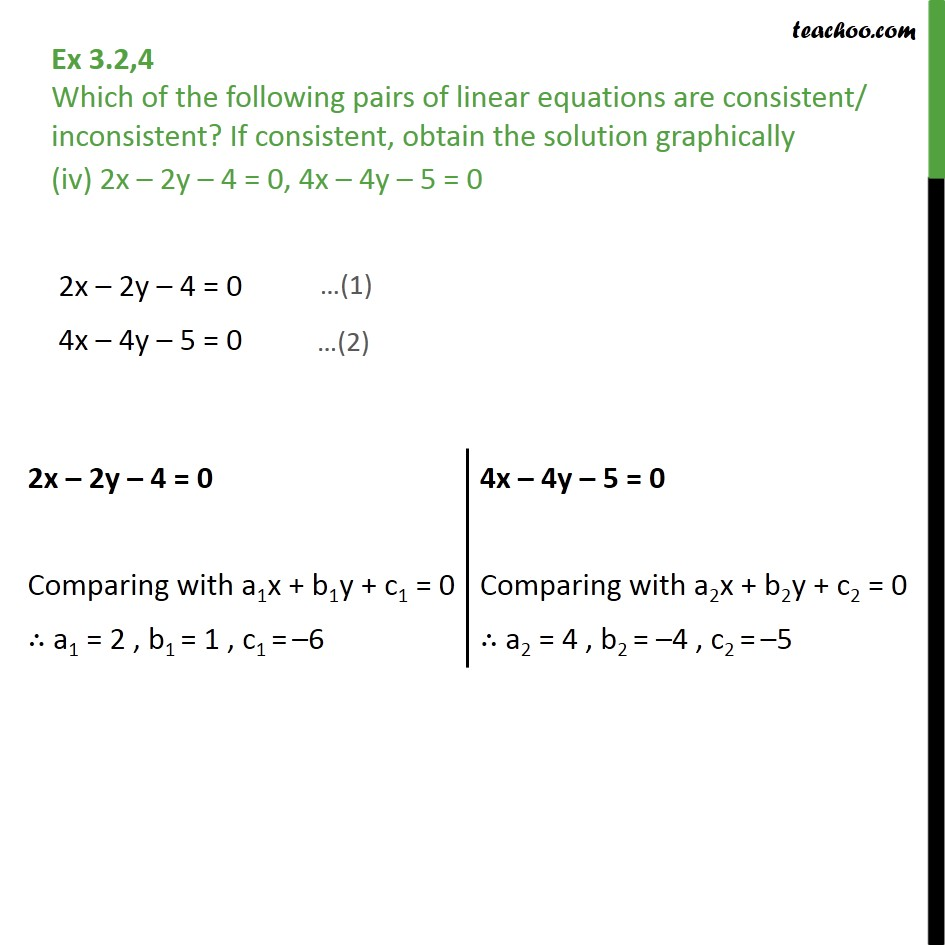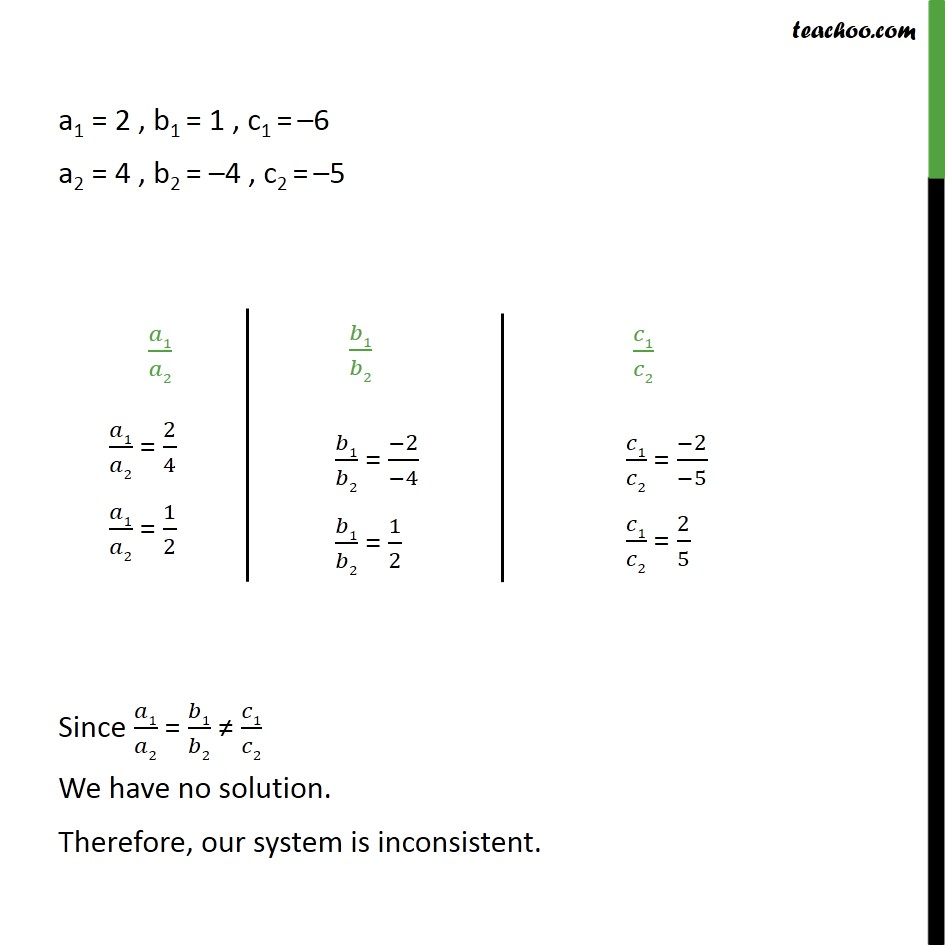1. Chapter 3 Class 10 Pair of Linear Equations in Two Variables
2. Serial order wise
3. Ex 3.2

Transcript

Ex 3.2,4 Which of the following pairs of linear equations are consistent/ inconsistent? If consistent, obtain the solution graphically x + y = 5, 2x + 2y = 10 x + y = 5 2x + 2y = 10 ∴ a1 = 1 , b1 = 1 , c1 = –5 & a2 = 2 , b2 = 2 , c2 = –10 Since 𝑎1/𝑎2 = 𝑏1/𝑏2 = 𝑐1/𝑐2 We have infinitely many solutions Therefore, our system is consistent. Now we solve our equations graphically Solving (1) x + y – 5 = 0 y = 5 − x Solving (2) 2x + 2y – 10 = 0 2y = 10 − 2x y = (10 − 2𝑥)/2 Ex 3.2,4 Which of the following pairs of linear equations are consistent/ inconsistent? If consistent, obtain the solution graphically (ii) x – y = 8, 3x – 3y = 16 x – y = 8 3x – 3y = 16 ∴ a1 = 1 , b1 = –1 , c1 = –8 & a2 = 3 , b2 = –3 , c2 = –16 Since 𝑎1/𝑎2 = 𝑏1/𝑏2 ≠ 𝑐1/𝑐2 We have no solution. Therefore, our system is inconsistent. Ex 3.2,4 Which of the following pairs of linear equations are consistent/ inconsistent? If consistent, obtain the solution graphically (iii) 2x + y – 6 = 0 , 4x – 2y – 4 = 0 2x + y – 6 = 0 4x – 2y – 4 = 0 a1 = 2 , b1 = 1 , c1 = –6 & a2 = 4 , b2 = –2 , c2 = –4 Since 𝑎1/𝑎2 ≠ 𝑏1/𝑏2 We have a unique solution. Therefore, our system is consistent. Solving (1) 2x + y – 6 = 0 y = 6 − 2x Solving (2) 4x − 2y – 4 = 0 2y = 4x – 4 y = (4𝑥 − 4)/2 Ex 3.2,4 Which of the following pairs of linear equations are consistent/ inconsistent? If consistent, obtain the solution graphically (iv) 2x – 2y – 4 = 0, 4x – 4y – 5 = 0 2x – 2y – 4 = 0 4x – 4y – 5 = 0 a1 = 2 , b1 = 1 , c1 = –6 a2 = 4 , b2 = –4 , c2 = –5 Since 𝑎1/𝑎2 = 𝑏1/𝑏2 ≠ 𝑐1/𝑐2 We have no solution. Therefore, our system is inconsistent.

Ex 3.2